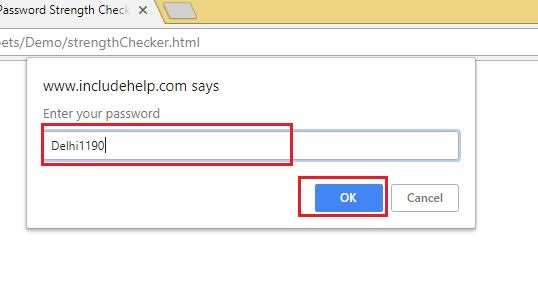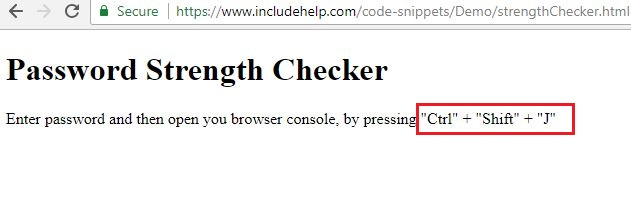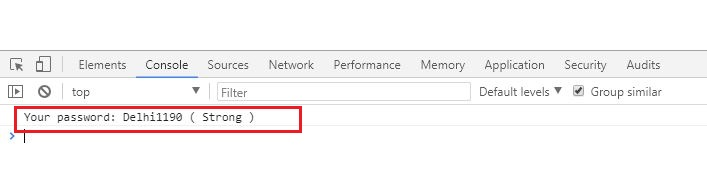# Password strength checker in JavaScript

In this JavaScript tutorial, we will learn how to implement password strength checker? This tutorial/article contains JavaScript code to check password strength.
Submitted by Abhishek Pathak, on June 15, 2018

If you have been a regular user of Web, you must have seen password strength functionality on many websites. Generally, when you go up to sign up or create your account, the website helps you to keep the secure password for your account by showing you your password strength.

With JavaScript, we can also do that very well. All you should be aware of is that what strength metrics you are considering. If you are planning to implement a password strength checker for your website, then you should consider this article.

JavaScript code:

```let strength = {
1: 'Very Weak',
2: 'Weak',
3: 'Medium',
4: 'Strong',
5: 'Very Strong'
};
```

First, we define strength values for our password strength checker. We have taken these values inside an object, so that we can directly check with the key the status, rather than using series of if-else.

JavaScript code:

```let strengthValue = {
'caps': false,
'length': false,
'special': false,
'numbers': false,
'small': false
};
```

Then we define strengthValue parameters for our tool. These are common metrics that you might have already seen, like if it includes capital letters, symbols, numbers etc. By default, the value is false since we won't count the false parameter in our strength value.

Now it's time to take input from the user and check the strength of their password.

JavaScript code:

```let password = prompt("Enter your password");

strengthValue.length = true;
}
```

We store this input in password variable and check it's length. Since many password checkers have length constraint, we take 8 here. If the length is greater than 8, then we make the length parameter true in strengthValue. Then we move on to check the string for strength metrics

JavaScript code:

```for(let index=0; index < password.length; index++) {
if(!strengthValue.caps && char >= 65 && char <= 90) {
strengthValue.caps = true;
} else if(!strengthValue.numbers && char >=48 && char <= 57){
strengthValue.numbers = true;
} else if(!strengthValue.small && char >=97 && char <= 122){
strengthValue.small = true;
} else if(!strengthValue.numbers && char >=48 && char <= 57){
strengthValue.numbers = true;
} else if(!strengthValue.special && (char >=33 && char <= 47) || (char >=58 && char <= 64)) {
strengthValue.special = true;
}
}
```

Here first we loop into every character of the password. Inside it, we get the char code of each character in the password. We need character code so that we can compare with other character's char code.

In the first if condition we check if the value of strengthValue.caps is false, since, if this metric is already satisfied, we don't want to check it again. Along with that, we check if the character code falls in between character code of A to Z. Likewise, we do for other metrics as well.

JavaScript code:

```let strengthIndicator = 0;
for(let metric in strengthValue) {
if(strengthValue[metric] === true) {
strengthIndicator++;
}
}

```

Finally, we check in to get the value of all the metrics. For this, we loop through every metric in strengthValue using for..in loop, that s used to traverse all the keys of an object.

Inside it, we simply check if the value of each metric is true. If it is, then increment the strengthIndicator value. And finally, we console out the value of the indicated value along with the password.

This was just a simple example, how you can create common functionality that we see every day with Javascript. See the demo here,

DEMO →

Output

1) It will prompt for password, enter the password and press okay.2) Press CTRL + SHIFT + J to open log.Hope you like the article. Share your thoughts in the comments below.